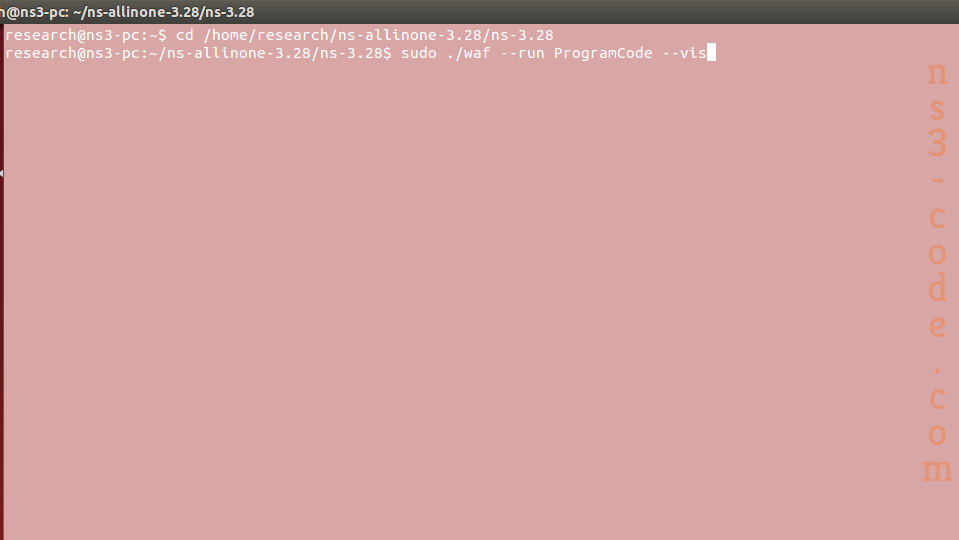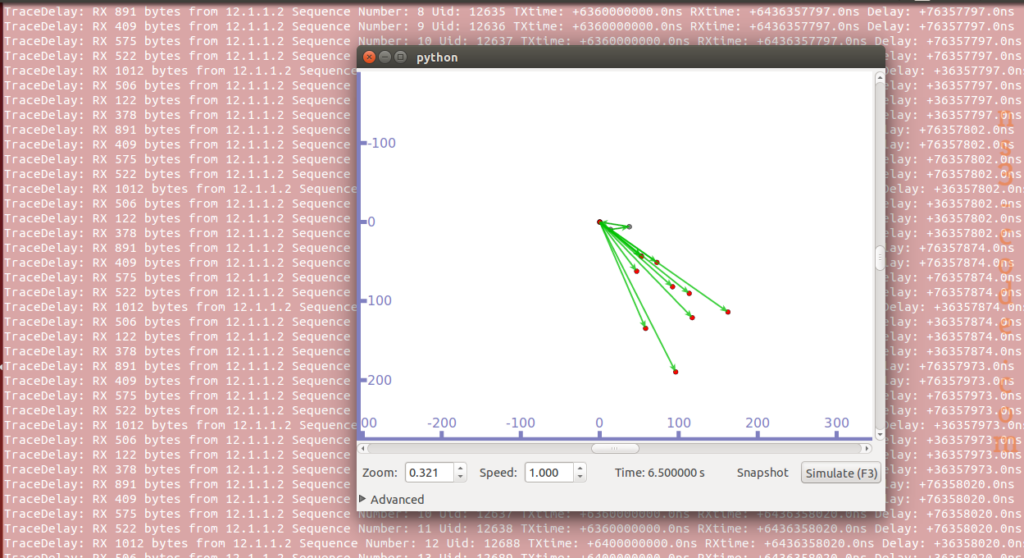## What is the Ns3 Program

In actual fact, the required steps involved in Ns3 program is considered as the simple process and through this article we have enlisted the required steps.

Stage: 1

As the first process, we have highlighted significant steps that are essential for the network simulator 3 program.

• Flow monitor
• Running the simulation
• Application configuration
• Mobility and positioning
• WiFi settings
• Channel configuration
• Node creation

Stage: 2

For your reference, we have highlighted some sample codes based on the network simulator 3 program.

• Mobility model
``` BSPosition = CreateObject (); BSPosition->SetPosition (Vector (1000, 0, 0)); bsNodes.Get (0)->AggregateObject (BSPosition); bsDevs.Add (dev); if (verbose) { wimax.EnableLogComponents (); // Turn on all wimax logging } for (int i = 0; i < nbSS; i++) { SSPosition[i] = CreateObject (); SSPosAllocator[i] = CreateObject (); Ptr xVar = CreateObject (); xVar->SetAttribute ("Min", DoubleValue ((i / 40.0) * 2000)); xVar->SetAttribute ("Max", DoubleValue ((i / 40.0 + 1) * 2000)); SSPosAllocator[i]->SetX (xVar); Ptr yVar = CreateObject (); yVar->SetAttribute ("Min", DoubleValue ((i / 40.0) * 2000)); yVar->SetAttribute ("Max", DoubleValue ((i / 40.0 + 1) * 2000)); SSPosAllocator[i]->SetY (yVar); SSPosition[i]->SetAttribute ("PositionAllocator", PointerValue (SSPosAllocator[i])); SSPosition[i]->SetAttribute ("Speed", StringValue ("ns3::UniformRandomVariable[Min=10.3|Max=40.7]")); SSPosition[i]->SetAttribute ("Pause", StringValue ("ns3::ConstantRandomVariable[Constant=0.01]")); ss[i] = ssDevs.Get (i)->GetObject (); ss[i]->SetModulationType (WimaxPhy::MODULATION_TYPE_QAM16_12); ssNodes.Get (i)->AggregateObject (SSPosition[i]);} ```
• Node creation
``` ssNodes.Create (nbSS); bsNodes.Create (1); Streamer_Node.Create (1); ASNGW_Node.Create (1); ```

Stage: 3

Finally, we have to run the program code in ns3 through the implementation of the below mentioned commands. Additionally, we have highlighted the results of the ns3 program code.

``` cd /home/research/ns-allinone-3.28/ns-3.28 sudo ./waf --run ProgramCode --vis ```Don’t worry if you’ve any uncertainties, because our technical experts are always equipped to help you.